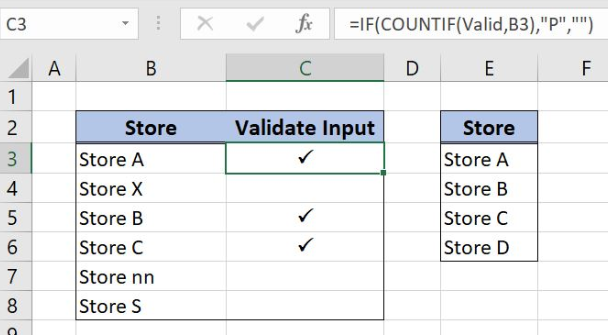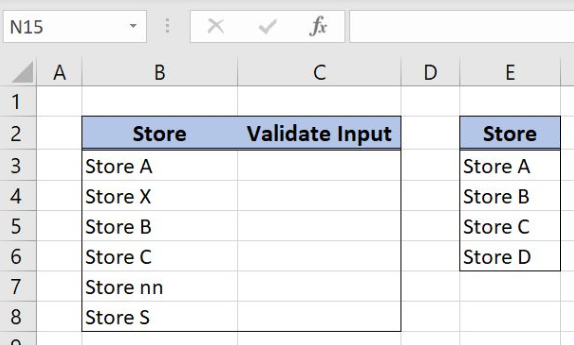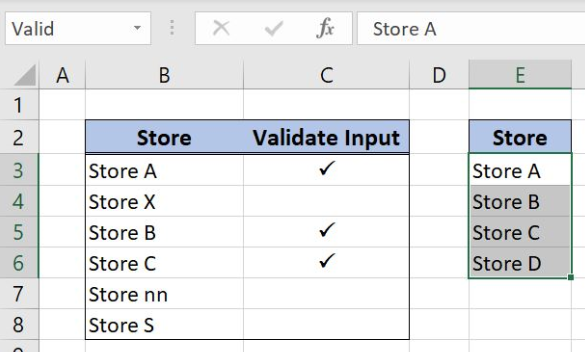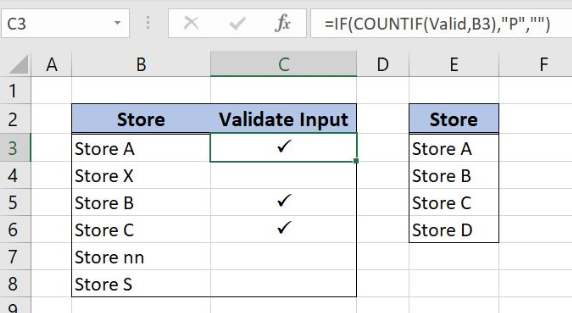Get instant live expert help with Excel or Google Sheets“My Excelchat expert helped me in less than 20 minutes, saving me what would have been 5 hours of work!”

#### Post your problem and you’ll get expert help in seconds.

Your message must be at least 40 characters
Our professional experts are available now. Your privacy is guaranteed.

# How to Validate Input with a Check Mark in Excel

Excel allows a user to validate input with check mark using IF and COUNTIF function. This step by step tutorial will assist all levels of Excel users in validating the inputs with check marks based on the data from another table.Figure 1. Validate store input with the check mark

## Syntax of the IF Formula

The generic formula for the IF function is:

`=IF(logical_test, value_if_true, value_if_false)`

The parameters of the IF function are:

• logical_test – a logical expression that we want to check
• value_if_true – a value which the function returns if a logical_test is TRUE
• value_if_false – a value which the function returns if a logical_test is FALSE.

## Syntax of the COUNTIF Formula

The generic formula for the COUNTIF function is:

`=COUNTIF(range, criteria)`

The parameters of the COUNTIF function are:

• range – range where we want to apply our criteria
• criteria – a criteria in criteria range which we want to count.

## Setting up Our Data to Validate Store Input with Check MarkFigure 2. Data that we will use in the example

Our first table consists of 2 columns: “Store” (column B) and “Validate Input” (column C) while the second one has one column “Store” (column E). The idea is to label the stores from column B that are matchable with the stores from the second table and to put the check mark in column C.

## Validate Stores with Check Mark Using IF and COUNTIF Functions

In our example, we want to check and mark the stores from the first table that exist in the second table using IF and COUNTIF functions. In order to make the formula more clear we will create a named range Valid for the cell range E3:E6.

To create a named range we should follow the steps:

• Select the cell range that should be named
• Click on the name box in Excel
• Write the name for the cell range and press enterFigure 3. Creating a named range Valid for the inputs validation

The formula looks like:

`=IF(COUNTIF(Valid,B3),"P","")`

The logical_test in the IF function is the formula COUNTIF(Valid,B3). The parameter value_if_true is the string “P” while the value_if_false is an empty string. In the COUNTIF function range is a named range Valid while the criteria is the cell B3.

To apply the function, we need to follow these steps:

• Select cell C3 and click on it
• Insert the formula: `=IF(COUNTIF(Valid,B3),"P","")`
• Press enter
• Drag the formula down to the other cells in the column by clicking and dragging the little “+” icon at the bottom-right of the cell
• Change a Font in column C to Wingdings 2 to display check marksFigure 4. Validate store inputs using IF and COUNTIF functions

COUNTIF function is checking if a store from column B exists in the column E. Result will be a number 1 if there is a store in column E and 0 if there is not. IF function interprets 0 value as a FALSE value and returns an empty string. If the result from the COUNTIF function is the number 1 IF function logical test will be TRUE and formula output will be a string “P”.

To convert a string “P” to the check mark, we just have to change a font in column C to the Wingdings 2. As a result, the check mark appears behind the stores that are matchable with the stores from the second table.

Most of the time, the problem you will need to solve will be more complex than a simple application of a formula or function. If you want to save hours of research and frustration, try our live Excelchat service! Our Excel Experts are available 24/7 to answer any Excel question you may have. We guarantee a connection within 30 seconds and a customized solution within 20 minutes.

### Did this post not answer your question? Get a solution from connecting with the expert.Another blog reader asked this question today on Excelchat:
Related blogs
Solution examplesCould you help me by explaining the IF function?
Solved by G. L. in 24 minsI have a question about Conditional Formatting. =IF('Sheet1'!A1="X",IF('Sheet1'!B1="Y", TRUE, FALSE),FALSE) the set the cell background to red. I would like to make it so that if Sheet1'!B1="Z" I set the back to blue. How can I achieve this.
Solved by B. F. in 39 minsI need to find out if the 2018 yearly sales goals were met if the yearly sales were \$25,000 or more using an IF logical function and to set the formula to return a value of YES if met, and NO if not
Solved by A. A. in 18 minsI filtered data in column A (it is labeled ID #) of a data set. On my next worksheet, column A is also the ID #, but it is a different data set. I want to filter the ID #'s the same for the two sheets, but how do I do that being that I am working with two different sets of data?
Solved by V. H. in 22 minsi want to create a very simple if function in a cell which is, if one cell is less than 1250, then the automatic value in the current cell is 250, dead easy, for someone who knows! Thank you.
Solved by A. H. in 14 mins## Subscribe to Excelchat.coAnother blog reader asked this question today on Excelchat: